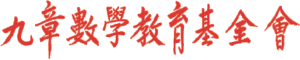首頁新聞區討論區檔案下載重要公告

 歷史公告

### 中學數學競賽

#### IYMC 2016

 主選單
 · 回首頁· 新聞區 · 討論區 · 檔案下載 · 網站連結 · 電子相薄 · 夥伴網站 · 精華文章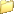討論區主頁10國中環球城市數學競賽2007秋季賽高級卷國中組第六題 限會員
 全部展開樹狀顯示 舊的在前 新的在前

Moderator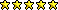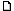環球城市數學競賽2007秋季賽高級卷國中組第六題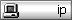6. 觀眾將n枚硬幣放在桌面上排成一列，每一枚硬幣朝上或朝下可隨觀眾喜好放置。觀眾也同時任意選1至n中的一個正整數，告訴魔術師的助手，接著魔術師的助手只能恰好選擇桌面上的一個硬幣將它翻面。魔術師不知道助手翻的是哪一個硬幣，但看一看桌面上的硬幣，竟能準確地猜出觀眾所選的數。魔術師與助手事先約定一些數學策略，使得魔術師能萬無一失地猜中。(a) 若對於某些n值魔術師能作到，試證對於2n值魔術師也能作到；（四分）(b) 請確定所有可能的n值。（五分）_________________孫文先 敬上2007-12-16 12:41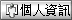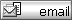betaveros
Just popping inRe: 環球城市數學競賽2007秋季賽高級卷國中組第六題(a)Let us assume that the magician and the assistant can perform for a number n. Then, the assistant has a way to change all possible settings of heads/tails into a setting that the magician will interpret as any given number. In other words, the duo have a way of interpreting any setting of heads/tails as a number from 1 to n, and changing any setting to a setting that reflects any given number.How to do it: assume the audience choose k.1. The assistant, given the setting of 2n coins, pairs each coin up with another one, to form n pairs.[O O] [O O] [O O] ...2. He interprets each pair as an individual coin by the following scheme: two heads or two tails = a head, a head and a tail either way = a tail. Let's call these new n coins "p-coins."3. He calculates the remainder of k / n, replacing 0 with n. Let this be x.4. He decides which p-coin to flip so as to make the p-coins show x. (He doesn't flip it or anything.)5. He calculates r: if k > n, r=1, otherwise r=0.6. He looks at the second coin of each p-coin, and computes how many heads are among this set of n coins, not p-coins.7. He finds the result of step 6's remainder when divided by 2. Call this s.8. He compares r (step 5) with s (step 7). If they are equal, then flip the first coin of the step 4 p-coin. If they are different, flip the second coin of the step 4 p-coin.How the magician interprets:1. Change the coins into p-coins by pairing them and valuing them with two alike = head and two unalike = tail like above, and calculate the p-coins' number like above.2. Count the heads in the second coins of each p-coin. If the number is odd, add n to the step 1 number. Otherwise, do not.This is the number.Proof: Clearly, the p-coins can represent a number of our choice, and they are designed so that flipping any coin in a p-coin will change the p-coin, so that 2n coins can show a number from 1 to n. Now, we want to show a number from 1 to 2n, so we have the second-coin head count. Flipping any second coin will change the count's parity, while flipping any first coin will preserve it. This bit of information can decide between x and n+x. Now, from flipping the p-coin we have narrowed the flippable coins to a first coin and a second coin. The head count narrows the flippable coins to either all first coins or all second coins, and clearly now exactly one coin will work.Q.E.D._________________This statement is false.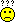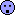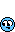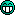Conclusion: "This statement is false" is not a statement.2007-12-17 20:37全部展開樹狀顯示 舊的在前 新的在前

 選擇討論區   九章數學基金會 ---------------- 讀書討論區 2012國際數學競賽專區   數學討論區 ---------------- 學習討論區 數學玩具討論區 小學 國中 高中 教學 其他 家長   九章數學教育基金會各項活動的感想與建議 ---------------- 參加小學數學競賽的感想與建議 參加中學數學競賽的感想與建議 參加九章數學俱樂部的感想與建議 參加奧林匹克訓培營的感想與建議 參加加拿大英文及數學夏令營的感想與建議 其它九章數學教育基金會活動的感想與建議   站內事務區 ---------------- 本站使用協助與建議區   九章數學愛好者聯誼會員專區 ---------------- 九章數學愛好者聯誼會員專區九章數學出版社、九章數學基金會版權所有本網頁各鍊結標題及鍊結內容歸原權利人所有Copyright 2000 ~2004九章數學出版社、九章數學基金會本網站內所有文字及資料版權均屬九章所有，未經書面同意之商業用途必究This web site was made with XOOPS, a web portal system written in PHP. XOOPS is a free software released under the GNU/GPL license.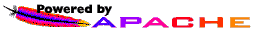Powered by XOOPS 1.3.10 © 2002 The XOOPS Project